Home > A2C > Chapter 9 > Lesson 9.3.2 > Problem9-155

9-155.
1. For each equation, find two solutions 0 < x < 2, which make the equation true. No calculator necessary. Homework Help ✎

1.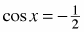2. tan x =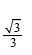3. sin x = 0

4. cos x =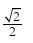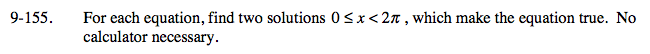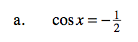$\text{cos}\left(\frac{\pi}{6}\right)=\frac{1}{2}$

What angle has the cosine of negative one half? Think of 30°, 60° right triangles.

x = 120° or 240°

$120\left(\frac{\pi}{180}\right)\,\,\,\,\,\,\,240\left(\frac{\pi}{180}\right)$

Now convert the angles into radians.

$\textit{x}=\frac{2\pi}{3},\,\frac{4\pi}{3}$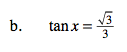See part (a).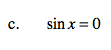If sin(x) = 0, y = 0

x = 0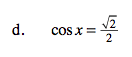Remember the side ratios of 45° right triangles.